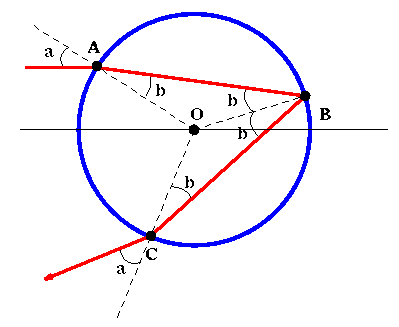# Simulating a Rainbow

In this section we will build on our previous work to compute the rainbow angle. If sunlight is traveling parallel to the earth, then the rainbow angle is the angle in the sky at which rainbows appear. Along the way we will examine what makes a rainbow. This simulation lets you follow rays of light as they are refracted (by Snell's law) and reflected in a raindrop.

In Figure 4 we depict a light ray hitting a water droplet at point A. Some of the light will be reflected and some of the light will enter the droplet. The light that enters will be refracted as discussed in the previous section. It then hits the other side of the droplet at point B where some of it will exit and the rest will reflect back. Finally at point C some of the light will exit and be seen by an observer.### Figure 4: Ideal path of a light ray through a spherical water droplet.

At the end of this page is a button. When you click on this button you will be able to experiment with "shooting" rays of light into a water droplet. The beam of light enters the water droplet at some height as measured from the droplet's center. This height is called the impact parameter. Each time you run the simulation, you will be told what angle the light leaves the water droplet (as measured from the horizontal). The angle at which light leaves the droplet is called the deflection angle. Answer the following questions using the information you gather from the simulation.

## Experiment #1

For the purpose of the first two experiments, you may ignore the "wavelength" of light rays. For best "experimental data," set the "wavelength" to be 700 nanometers (red light). (We will look at the effect of wavelength later.)

## Experiment #2

The next activity will help you answer the question "When will incoming light be focussed most intensely?" In other words, when will a range of incoming rays all leave the droplet at approximately the same angle?
• Send in 3 incoming beams of light at:
• impact parameters 0.05, 0.1, 0.15
• impact parameters 0.5, 0.55, 0.6
• impact parameters 0.75, 0.8, 0.85
• Sketch the results
• For which set of impact parameters are the outgoing rays the most concentrated? The most diffuse?
• If an incoming ray has impact parameter w, we define D(w) to mean the deflected angle of that light ray. Use the results of the previous experiment to complete the chart you were given.

## Experiment #3

Repeat Experiment #1 with the wavelength of the incoming light rays set to 400 nanometers (violet light).
• How does the angle of minimum deflection change?
The color of light corresponds to its wavelength. Light with a wavelength of 400 nanometers is violet; a wavelength of 450 nanometers is blue. Light with a wavelength of 525 nanometers appears green, 580 nanometers is yellow, 625 nanometers corresponds to orange, and 700 nanometers is red.
• Conjecture how the minimum angle of deflection varies according to the wavelength of light. Specifically, as the wavelength of light decreases, does the minimum angle of deflection increase or decrease? (Make sure your conjecture is supported by your results from Experiment #1 and the first portion of Experiment #3.)
"White" light is composed of light of all wavelengths. Therefore a "true" simulation of light passing through a water droplet is to send in several rays of differing wavelengths at the same impact parameter and to see how these rays disperse into a rainbow.

Send in 5 incoming beams of light at impact parameters 0.9. The wavelengths of the incoming beams should be 400, 450, 550, 600, and 700 nanometers.

• What are the corresponding angles of the outgoing rays?

Next:Analysis
Previous:Refraction